# 分享敏感词过滤实现

## 词库的准备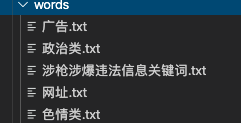## DFA算法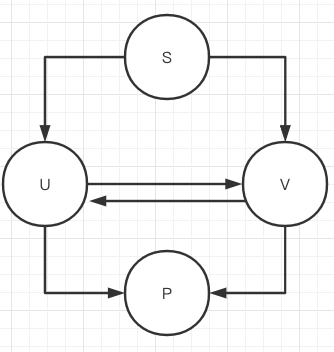### 例子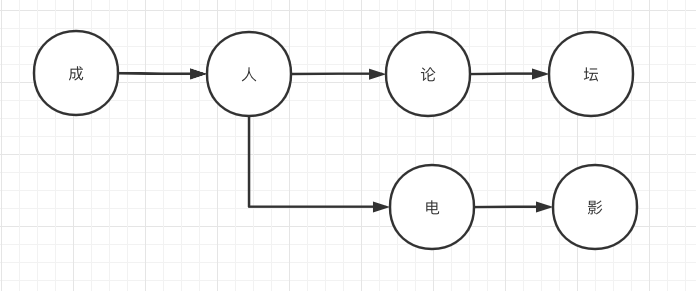### 具体流程

#### 1.清洗数据

``````*-`J情成&^人电影在**\$#线观看
``````

``````J情成人电影在线观看
``````

#### 2.字符穷举

``````J
J情
J情成
J情成人
J情成人电
J情成人电影
J情成人电影在
J情成人电影在线
J情成人电影在线观
J情成人电影在线观看
``````

#### 检测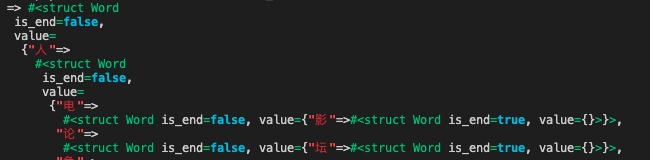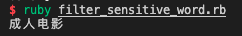## 源码

``````require “set"
require “pry”
require ‘active_support/core_ext’

Word = Struct.new(:is_end, :value)

class FilterSensitiveWord
attr_accessor :words
MIN_MATCH_TYPE = 1
MAX_MATCH_TYPE = 2

def initialize()
@hash = Hash.new
end

@words = Set[]
f = File.open(file_path, “r”)
f.each_line do |line|
end
f.close
to_hash(@words)
end

def to_hash(words)
words.map do |word|
word_hash = @hash
word.strip.chars.to_a.map.with_index do |c,index|
if word_hash[c].blank?
if word.strip.length - 1 == index
word_hash[c] = Word.new(true,Hash.new)
else
word_hash[c] = Word.new(false,Hash.new)
word_hash = word_hash[c].value
end
else
word_hash = word_hash[c].value
end
end
end
@hash
end

def check_sensitive_word(txt, index, match_tpye)
match_flag = 0
word_hash = @hash
flag = false
txt.each do |w|
if word_hash[w].blank?
break
else
match_flag = match_flag + 1
if word_hash[w].is_end
flag = true
if match_tpye == MIN_MATCH_TYPE
break
end
else
word_hash = word_hash[w].value
end
end
end
unless flag
match_flag = 0
end
match_flag
end

def check(text,match_tpye)
words = []
skip_len = 0
text = text.strip.gsub(/[`~!@#\$^&*()=|{}':;',\\\[\]\.<>\/?~！@#￥……&*（）——|{}【】'；：""'。，、？]/,'')
if text.present?
text.chars.each_with_index do |c, i|
if skip_len > i
next
end
len = check_sensitive_word(text.chars.drop(i), i, match_tpye)
if len > 0
words.push text[i, len]
skip_len = i + len - 1
end
end
end

words
end

end

\$filter_sensitive_word = FilterSensitiveWord.new()
puts \$filter_sensitive_word.check("*-`J情成&^人电影在**\$#线观看",1)

``````

## 参考资料

• java 敏感词之 DFA 算法(Tire Tree 算法)
• java实现敏感词过滤（DFA算法）